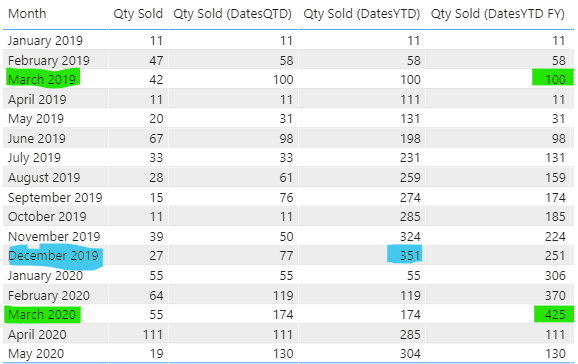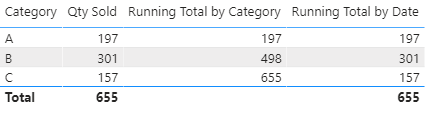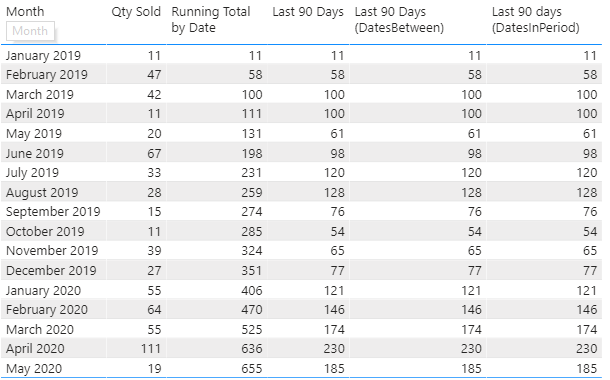top of page
Search

# DAX Time Intelligence Part 2: Till Date AggregationsIn the previous article, we have learned a few functions on time intelligence. This article covers time intelligence functions on doing date-based totals like a year-to-date, quarter-to-date, month-to-date.

We are using the same data file as used in the previous article.

Note: I have used variables extensively in the article. Visit this article to know more details on using variables in DAX:

DAX: Using Variables

## Understanding Periods to Date (DATESYTD, DATESQTD & DATESMTD)

Using FILTER function, this is how we can calculate MTD total (in this case, Qty Sold):

``` Test FILTER MTD =
VAR _LatestDate = MAX(ftCalendar[Date])

// Filter the date range
VAR _Filter =
FILTER(
ALL(ftCalendar[Date]),
ftCalendar[Date] <= _LatestDate
&& YEAR(ftCalendar[Date]) = YEAR(_LatestDate)
&& MONTH(ftCalendar[Date]) = MONTH(_LatestDate)
)

VAR _Calc =
CALCULATE(
[Qty Sold],
_Filter
)

RETURN
_Calc
```

Essentially, in the FILTER statement, we are telling DAX that:

· Remove any existing filter context applied (using ALL statement)

· Return a range of dates where:

o the last date in the table is less than or equal to the max date of the context

o Year of the dates returned is equal to the year of the max date of the context

o The month of the dates returned is equal to the month max date of the context

So, if the date selected is 20-May-2020, then it returns all the dates which are in the current month and current year and is less than or equal to 20th May 2020. In short, all dates from 1st May to 20th May 2020.

DAX offers specific time intelligence functions to calculate month-till-date, quarter-till-date & year-till-date totals. They are DATESMTD, DATESQTD, and DATESYTD, respectively. Each of these functions returns a table with a range of dates. It gives the same results using the FILTER function in the expression above.

### Using DATESMTD

Using DATESMTD, we can re-write the above expression:

``` Qty Sold (DatesMTD)RT =
CALCULATE(
[Qty Sold],
DATESMTD(ftCalendar[Date])
)
```

The above expression creates the following output:Notice it adds a row in the visual for all the dates in the calendar table, even for the days where we do not have data for Qty sold. We can modify the formula to update the output:

``` Qty Sold (DatesMTD) =
IF(
//checks for the records available in the data table
HASONEVALUE(dtTable[Date]),
CALCULATE(
[Qty Sold],
DATESMTD(ftCalendar[Date])
)
)
```

We can read the above statement: if we have a record available for a given date in the data table, then calculate the month-till-date sum of Quantity sold.### Using DATESQTD & DATESYTD

Like DATESMTD, we have DATESQTD and DATESYTD. It returns a range of dates starting from the first day of the quarter and the calendar year, respectively.

``` Qty Sold (DatesQTD) =
CALCULATE(
[Qty Sold],
DATESQTD(ftCalendar[Date])
)

Qty Sold (DatesYTD) =
CALCULATE(
[Qty Sold],
DATESYTD(ftCalendar[Date])
)
```### Financial Year with DATESYTD

DATESYTD function offers an additional argument for selecting the financial year. For example, the financial year for India is April to Mar. We can modify the formula to get the YTD according to financial year:

``` Qty Sold (DatesYTD FY) =
CALCULATE(
[Qty Sold],
DATESYTD(ftCalendar[Date], "3/31")
)```

DATESYTD takes the year-end month and date as the second argument. In this example, our financial year ends on 31st March. Hence we have added “3/31”.## Calculating Running Total

Apart from MTD, QTD, and YTD calculations, we sometimes require calculating the running total like running total for the selected date range:We use the following measure for this:

``` Running Total by Date =
VAR _LatestDate = MAX(ftCalendar[Date])

// Filter the date range
VAR _Filter =
FILTER(
ALLSELECTED(ftCalendar[Date]),
ftCalendar[Date] <= _LatestDate
)

VAR _Calc =
CALCULATE(
[Qty Sold],
_Filter
)

RETURN
_Calc
```

We have used ALLSELECTED to take the minimum date of the defined date range as the start date. In the table above, we have the start date as 1st January 2019 for all the months. Hence, for March 2019, it is calculating Qty Sold for the date range 1st January 2019 to 31st March 2019.

## Running Total for Categorical Data

Calculating running total by the categories is like we did for dates. We modify the measure and replace date by category:

``` Running Total by Category =
//declaring the current category value
VAR _Category = SELECTEDVALUE(dtTable[Category])

//filtering the table by each category
VAR _Filter =
FILTER(
ALLSELECTED(dtTable[Category]),
dtTable[Category] <= _Category
)

//calculating the running total
VAR _RT =
CALCULATE(
[Qty Sold],
_Filter
)

RETURN
_RT
```Note that Running Total by Date is not returning the desired result as the filter context is different in this case.

More on Running Totals

## Totals for Specific Periods

Just like running totals by till date, we can define the start date and end date to define the date range for the calculation:

``` Last 90 Days =
VAR _LatestDate = MAX(ftCalendar[Date])
VAR _Duration = 90
VAR _StartDate = _LatestDate - _Duration

// Filter the date range
VAR _Filter =
FILTER(
ALLSELECTED(ftCalendar[Date]),
ftCalendar[Date] >= _StartDate
&& ftCalendar[Date] <= _LatestDate
)

VAR _Calc =
CALCULATE(
[Qty Sold],
_Filter
)

RETURN
_Calc
```

### Using DATESBETWEEN

Alternatively, we can write this using DATESBETWEEN:

``` Last 90 Days (DatesBetween) =
VAR _LatestDate = MAX(ftCalendar[Date])
VAR _Duration = 90
VAR _StartDate = _LatestDate - _Duration

VAR _Calc =
CALCULATE(
[Qty Sold],
DATESBETWEEN(ftCalendar[Date],_StartDate,_LatestDate)
)

RETURN
_Calc
```

DATEBETWEEN returns a table with dates between two dates. It takes three arguments for calculation:

· The column for the date filter

· Start Date

· End Date### Using DATESINPERIOD

We can further simplify the expression by using DATESINPERIOD:

```  Last 90 days (DatesInPeriod) =
VAR _LatestDate = MAX(ftCalendar[Date])

VAR _Calc =
CALCULATE(
[Qty Sold],
DATESINPERIOD(ftCalendar[Date],_LatestDate,-91,DAY)
)

RETURN
_Calc
```

DATESINPERIOD returns a table with a list of dates from a given period. It takes the following arguments for calculation:

• The column for the date filter

• Start Date

• Number of Intervals (It takes integers as inputs. Negative values go back in the past, positive values in future)

• Interval (DAY, MONTH, QUARTER, YEAR)

In the measure above, we are asking DAX to take the date column of the ftCalendar table into consideration. From the max date in the selection, go to 91 days in the past.Note: We have used 91 instead of 90 in the argument because DATESINPERIOD includes the start date into consideration, hence effectively, it moves 89 periods in the past. For example, if we select 20th May 2020, it returns a table with a date starting from 22nd January 2020. Whereas, we need to start our calculation from 21st January 2020.

@imVivRan

bottom of page